Test: Inductance - 2

# Test: Inductance - 2

Test Description

## 15 Questions MCQ Test Network Theory (Electric Circuits) | Test: Inductance - 2

Test: Inductance - 2 for Electrical Engineering (EE) 2023 is part of Network Theory (Electric Circuits) preparation. The Test: Inductance - 2 questions and answers have been prepared according to the Electrical Engineering (EE) exam syllabus.The Test: Inductance - 2 MCQs are made for Electrical Engineering (EE) 2023 Exam. Find important definitions, questions, notes, meanings, examples, exercises, MCQs and online tests for Test: Inductance - 2 below.
Solutions of Test: Inductance - 2 questions in English are available as part of our Network Theory (Electric Circuits) for Electrical Engineering (EE) & Test: Inductance - 2 solutions in Hindi for Network Theory (Electric Circuits) course. Download more important topics, notes, lectures and mock test series for Electrical Engineering (EE) Exam by signing up for free. Attempt Test: Inductance - 2 | 15 questions in 45 minutes | Mock test for Electrical Engineering (EE) preparation | Free important questions MCQ to study Network Theory (Electric Circuits) for Electrical Engineering (EE) Exam | Download free PDF with solutions
 1 Crore+ students have signed up on EduRev. Have you?
Test: Inductance - 2 - Question 1

### The SI unit of circuit element inductance is:

Detailed Solution for Test: Inductance - 2 - Question 1

Concept:

• Induction: It is the magnetic field that is proportional to the rate of change of the magnetic field.
• This definition of induction holds for a conductor. Induction is also known as inductance.
• L is used to represent the inductance and Henry is the SI unit of inductance.
• 1 Henry is defined as the amount of inductance required to produce an emf of 1 volt in a conductor when the current change in the conductor is at the rate of 1 Ampere per second.
• Following are the factors that affect the inductance:
(i) The number of turns of the wire used in the inductor.
(ii) The material used in the core.
(iii) The shape of the core.
• Electromagnetic Induction: The law was given by Faraday which states that by varying the magnetic flux electromotive force is induced in the circuit.
• Inductance: It can be defined as the electromotive force generated to oppose the change in current at a particular time duration.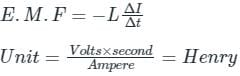Test: Inductance - 2 - Question 2

### A pure inductor of 20.0 mH is connected to a source of 220 V. Find the RMS value of current in the circuit, if the frequency of the source is 50 Hz.

Detailed Solution for Test: Inductance - 2 - Question 2

Concept:

Inductive reactance:

• The inductive reactance is the opposition offered by the inductor in an AC circuit to the flow of ac current.
• Its SI unit is ohm(Ω).
• The inductive reactance is given as,

⇒ XL = 2πfL

Where f = frequency of ac current and L = self - inductance of the coil

Impedance:

• Impedance is essentially everything that obstructs the flow of electrons within an electrical circuit.
• For a pure inductor, the inductive reactance is equal to the impedance.

AC voltage applied to an inductor:

• When an AC voltage is applied to an inductor, the current in the circuit is given as,

⇒ I = V / XL

• In a pure inductor circuit, the current reaches its maximum value later than the voltage by one-fourth of a period.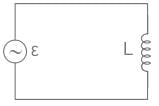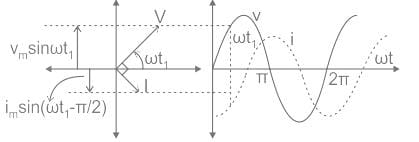Calculation:

Given Vrms = 220 V

L = 20 mH = 20 × 10-3 H

f = 50 Hz

Inductive reactance is given as,

⇒ XL = 2πfL

⇒ XL = 2π × 50 × 20 × 10-3

⇒ XL = 6.28 Ω

When an AC voltage is applied to an inductor, the current in the circuit is given as,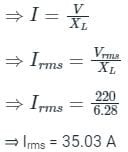Test: Inductance - 2 - Question 3

### An air-cored solenoid has 100 turns and is 1 m long. If the area of the cross section is 100 cm2, what will be its self-inductance (in μH)?

Detailed Solution for Test: Inductance - 2 - Question 3

Concept:

Self-inductance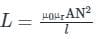Here, A = Area

N = Number of turns

l = Length of coil

μ0 = absolute permeability of material

μr = relative permeability of material

Calculation:

N = 100, l = 1 m,

A = 100 cm2 = 100 cm × cm = 100 × 10-2 m × 10-2 m = 100 × 10-4 m2

μ∘ = 4 π × 10-7, μr = 1 (Air)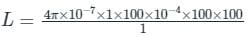40 π μH

Test: Inductance - 2 - Question 4

Which of the following has Henry as their SI unit?

Detailed Solution for Test: Inductance - 2 - Question 4

Concept:

• Inductor: The coil which stores magnetic energy in a magnetic field is called an inductor.
• Inductance: The property of an inductor that causes the emf to generate by a change in electric current is called the inductance of the inductor.
• The SI unit of inductance is Henry (H).
Test: Inductance - 2 - Question 5

In inductive circuit, when inductance (L) or inductive reactance (XL) increases, the circuit current

Detailed Solution for Test: Inductance - 2 - Question 5

Concept:

The current in an inductive circuit is given by: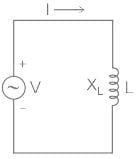I = V/XL

where I = Current

V = Voltage

XL = Inductive reactance

Explanation:

• XL is inversely proportional to the current.
• Hence, with an increase in the inductive reactance, the current decreases.
Test: Inductance - 2 - Question 6

Basic unit of inductance is ______.

Detailed Solution for Test: Inductance - 2 - Question 6

Key Points

• Mutual Inductance is the interaction of one coil's magnetic field on another coil as it induces a voltage in the adjacent coil.
• It is the basic operating principle of the transformer, motors, generators and many other electrical components that interact with another magnetic field.
• The mutual inductance between the two coils can be greatly increased by positioning them on a common soft iron core or by increasing the number of turns.
Test: Inductance - 2 - Question 7

When a dc voltage of 60 V is applied across a solenoid, an electric current of 5 A flows through it. When the dc voltage is replaced by the ac voltage of the angular frequency 400 rad/s, the electric current is reduced to 3A. What is the inductance of solenoid?

Detailed Solution for Test: Inductance - 2 - Question 7

Concept:

Solenoid:

A type of electromagnet that generates a controlled magnetic field through a coil wound into a tightly packed helix.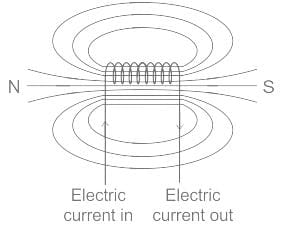• The uniform magnetic field is produced when an electric current is passed through it.
• The magnetic field inside a solenoid does not depend on the diameter of the solenoid.
• The field inside is constant.

Calculation:

Given: Vdc = 60 V, Idc = 5 A

Solenoid equivalent is given by series RL circuit.

Now by DC supply, the inductor behaves as a short circuit at a steady state.

Vdc = Idc × R = 5 × R = 60

R = 12 Ω

Now, when AC supply is given, Current Iac = 3 A at ω = 400 rad/sec

Equivalent impedance(Z) = V/I = 60/3 = 20Ω

Therefore,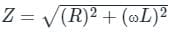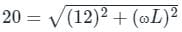ωL = 16 Ω

L = 16/400 = 0.04 H

Test: Inductance - 2 - Question 8

Two coils with self-inductance of 100 mH and 200 mH and mutual inductance of 50 mH are connected in parallel aiding order. What will be the total inductance of the combination?

Detailed Solution for Test: Inductance - 2 - Question 8

Concept:

The equivalent inductance of series aiding connection is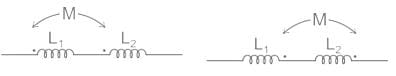L = L1 + L2 + 2M

The equivalent inductance of series opposing connection is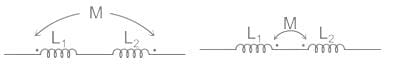L = L1 + L2 – 2M

Equivalent inductance of parallel aiding connection is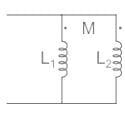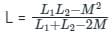Equivalent inductance of parallel opposing connection is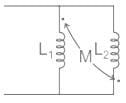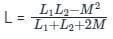Calculation:

Given,

L1 = 100 mH

L2 = 200 mH

M = 50 mH

Inductance of parallel aiding connection is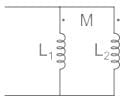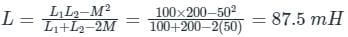Test: Inductance - 2 - Question 9

The inductance of a single layer coil of 50 turns is 5 mH. If the no. of turns is doubled, the inductance of the coil will become ______

Detailed Solution for Test: Inductance - 2 - Question 9

Concept:

The formula for inductance of a coil is

L = μN2A / l

N is no. of turns

A is the cross-sectional area

l length of the solenoid

L ∝ N2 / l

L2L= (N2 / N1)2 x  l1l2

Calculation:

Given:

L1 = 5 mH, N1 = 50 turns

Now, The number of turns is doubled i.e.

N2 = 2N1 = 100

l= l1 = l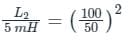L2 = 20 mH

Test: Inductance - 2 - Question 10

The _______ the permeability of the core material, the _______ will be the inductance.

Detailed Solution for Test: Inductance - 2 - Question 10

Concept:

Inductance is the property of a component that opposes the change of current flowing through it and even a straight piece of wire will have some inductance.

Therefore, the below equation will give inductance L as being proportional to the number of turns squared N2

The inductance of a coil of wire is given by,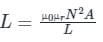Where N is the number of turns

A is the cross-sectional area

L is the length of the solenoid.

μr is the relative permeability of the core material

From the above relation, we observe that the inductance is directly proportional to the permeability of the material, i.e. higher the permeability of the core material, higher will be the inductance.

Test: Inductance - 2 - Question 11

An iron-cored coil has an inductance of 4 H. If the reluctance of the flux path is 100 AT/Wb, then the number of turns in the coil is:

Detailed Solution for Test: Inductance - 2 - Question 11

Concept:
Self-Inductance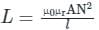Here, A = Area

N = Number of turns

l = Length of coil

magnetic flux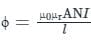Reluctance s = N2 / L.......(i)

Calculation:

L = 4 H, s = 100 AT / Wb

From equation (i)

N = √4×100

20

Test: Inductance - 2 - Question 12

A 500 W discharge lamp takes a current of 4 A at unity p.f. Find the inductance of a choke required to enable the lamp to work on 250 V, 50 Hz main.

Detailed Solution for Test: Inductance - 2 - Question 12

Concept :

Choke coil (or ballast) is a device having high inductance and negligible resistance. It is used to control current in ac circuits and is used in fluorescent tubes. The power loss in a circuit containing choke coil is least.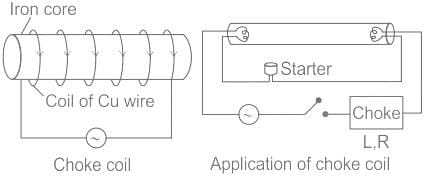(1) It consist of a Cu coil wound over a soft iron laminated core

(2) Thick Cu wire is used to reduce the resistance (R) of the circuit

(3) Soft iron is used to improve inductance (L) of the circuit

(4) The inductive reactance or effective opposition of the choke cost is given by XL = 2πfL

(5) For an ideal choke coil r= 0, no electric energy is wasted i.e. average power P=0

6) In actual practice choke coil is equivalent to a R-L circuit.

(7) Choke coil for different frequencies are made by using different substances in their core.

For low frequency L should be large thus iron core choke coil is used. For high frequency ac circuit, L should be small, so air cored choke coil is used.

Calculations:

P = IR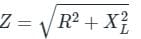XL = 2 π f L

L= XL / 2πf

Given

P = 500 W

I = 4 A

V = 250 V

f = 50 Hz

pf  = unity

R = P / I2  = 500 / 16 = 31.25 Ω

Z =  V / I = 250 / 4 = 62.5 Ω

XL = 54.125 Ω

L = XL  / (2π f) = 0.1732 H

Test: Inductance - 2 - Question 13

Self-induction is sometimes analogously called:

Detailed Solution for Test: Inductance - 2 - Question 13

Self induction:

• The self inductance is defined as the property by virtue of which coil opposes any change in the current flowing through it.
• Due to this self inductance, when the current in the coil is increased, the self induced EMF in it will oppose this increase by acting in the direction opposite to that of the applied EMF.
• Similarly, if the current in the coil is decreased, he self induced EMF will tend to keep the current at its original value by acting in the same direction as the applied EMF.
• Thus, any change in the current  through the coil is opposed due to its self inductance.
• Hence, self-inductance is sometimes analogously called electrical inertia or electromagnetic inertia.
Test: Inductance - 2 - Question 14

If L1 and L2 are the inductances of coil-1 and coil-2, then the total inductance of the magnetic circuit will be represented as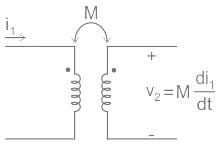Detailed Solution for Test: Inductance - 2 - Question 14

Concept:

• When inductors are connected in series so that the magnetic field of one links with the other, the effect of mutual inductance either increases or decreases the total inductance depending upon the amount of magnetic coupling.
• The effect of this mutual inductance depends upon the distance apart of the coils and their orientation to each other.
• Mutually connected series inductors can be classed as either “Aiding” or “Opposing” the total inductance.

Mutually Aiding: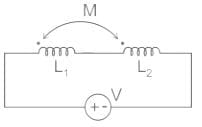Leq = L1 + L2 + 2M

L : self-inductance

M: Mutual inductance

Mutually Opposing: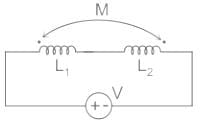Leq = L1 + L2 – 2M

Test: Inductance - 2 - Question 15

An iron cored coil with 1000 turns generates a magnetic flux of 500 μwb in the core while carrying an electric current of 50 A. What will be the self-inductance of the coil?

Detailed Solution for Test: Inductance - 2 - Question 15

Concept:

Self-inductance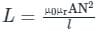Here, A = Area

N = Number of turns

l = Length of coil

magnetic flux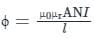Calculation:

N= 1000, ϕ = 500 μwb, I = 50 A

Rl = reluctance

ϕ = (1000 × 50 ) / Rl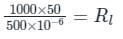R= 108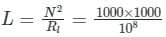L = 0.01 H

## Network Theory (Electric Circuits)

23 videos|63 docs|60 tests
 Use Code STAYHOME200 and get INR 200 additional OFF Use Coupon Code
Information about Test: Inductance - 2 Page
In this test you can find the Exam questions for Test: Inductance - 2 solved & explained in the simplest way possible. Besides giving Questions and answers for Test: Inductance - 2, EduRev gives you an ample number of Online tests for practice

## Network Theory (Electric Circuits)

23 videos|63 docs|60 tests